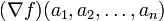Relation between gradient vector and partial derivatives

Statement

Version type Statement
at a point, in multivariable notation Suppose$f$ is a real-valued function of$n$ variables$x_1,x_2,\dots,x_n$. Suppose$(a_1,a_2,\dots,a_n)$ is a point in the domain of$f$ such that the gradient vector of$f$ at$(a_1,a_2,\dots,a_n)$, denoted$(\nabla f)(a_1,a_2,\dots,a_n)$, exists. Then, the partial derivatives of$f$ with respect to all variables exist, and the coordinates of the gradient vector are the partial derivatives. In other words:$(\nabla f)(a_1,a_2,\dots,a_n) = \langle f_{x_1}(a_1,a_2,\dots,a_n), f_{x_2}(a_1,a_2,\dots,a_n), \dots f_{x_n}(a_1,a_2,\dots,a_n)\rangle$
generic point, in multivariable notation Suppose$f$ is a real-valued function of$n$ variables$x_1,x_2,\dots,x_n$. Then, we have$(\nabla f)(x_1,x_2,\dots,x_n) = \langle f_{x_1}(x_1,x_2,\dots,x_n), f_{x_2}(x_1,x_2,\dots,x_n), \dots f_{x_n}(x_1,x_2,\dots,x_n)\rangle$.
Equality holds wherever the left side makes sense.
generic point, point-free notation Suppose$f$ is a function of$n$ variables$x_1,x_2,\dots,x_n$. Then, we have$\nabla f = \langle f_{x_1}, f_{x_2}, \dots f_{x_n} \rangle$. Equality holds wherever the left side makes sense.

Note on continuous partials

For further information, refer: Continuous partials implies differentiable

This says that if all the partial derivatives of a function are continuous at and around a point in the domain, then the function is in fact differentiable, hence the gradient vector is described in terms of the partial derivatives as described above.

In particular, if all the partials exist and are continuous everywhere, the gradient vector exists everywhere and is given as described above.

Note that this is significant because, a priori (i.e., without checking continuity), knowledge of the partials tells us what the gradient vector should be if it exists, but it doesn't tell us whether the gradient vector does exist. Continuity helps bridge that knowledge gap.